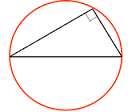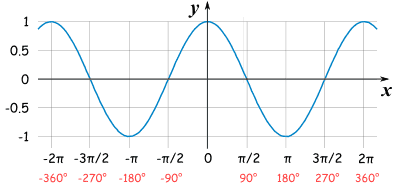# core 2

• Created by: courteny
• Created on: 27-04-16 12:02

core two

1 of 12

## rules of logarithmsLOGaN=X is the same as a^x=n

2 of 12

## geometric proof

sn= a + ar + ar^2...........ar^n-2 + ar^n-1

rsn=     ar + ar^2...........ar^n-1 + ar^n-1 + ar^n

sn-rsn =  a-ar^n

sn(1-r)= a(1-r^n)                        5% increase  equals 1.05

sn = a(1-r^n)                               5% decrease equals 95

--------

1-r

sum to infinity = a/1-r                a=1st number        r=common ratio

3 of 12

area of sector  =  angle at center         length of arc  = angle of center

total area             total angle             circumference    total angle of

area of sector   =             0                                           center

pie x r^2                      2x pie                      L         0

area of sector =              0r^2                  2xpiexr    2x pie

2                   length of arc = r0

4 of 12

## algebra and functions

if f(a)is a polynomial and f(a)=0 then (x-a) is a factor

if f(a) is a polynomial and f(b/a)=0 the (ax-b) is a factor

if a polynomial is divided by (ax-b)then the remainder is is f(b/a)

5 of 12

## the sine and cosine rule

a       =       b

sinA         sin B

A^2=B^2+C^2-2BCCOSA

cosA = b^2+c^2-a^2

2bc

area = 1/2absinC

6 of 12

## coordinate geometry in the (x,y)plane

midpoint = (x1+x2  ,  y1+y2)

2            2

distance between two points

d^2=(x2-x1)^2+(y2-y1)^2

equation of a circle is (x-a)^2+(y-b)^2=r^2

angle inside a semicircle is a right angle7 of 12

## the binomial expansion8 of 12

## graphs of trigonometric identitiescos graph                                          sin graph

9 of 12

## differentiation

increasing function f'(x) > 0

decreasing function f'(x)<0

coordinates of a stationary point find f'(x) find value of x then find y

f''(x)<0 maximum point

f''(x0>0 minimum point

10 of 12

## trigonometrical identies and simple equations11 of 12

## integration12 of 12